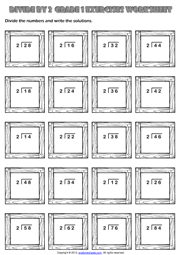Home Maths Main Page

# Divide by 2 Maths Exercise Worksheet With Two Digits

An easy division exercise maths worksheet without remainders for grade 1 (first grade) students and kids with wooden frames theme. Divide the numbers by two and write the solutions. (Long Division Worksheet for kids)Next Worksheet Grade 1 Division Main Page Home